### Home > CC4 > Chapter 10 > Lesson 10.1.3 > Problem10-54

10-54.
1. Solve for all possible values for x in each equation. Homework Help ✎

1. – 2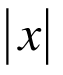= – 8

2.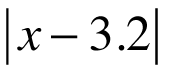= 4.7

3.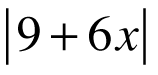= 4

4.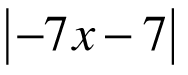= 1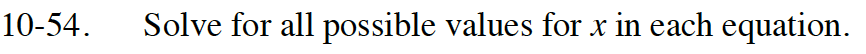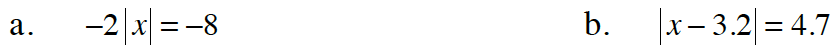Divide both sides by -2 to isolate the variable in the absolute value.

Either x −3.2 = 4.7 or x −3.2 = -4.7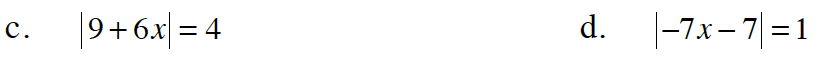Either 9 + 6x = 4 or 9 + 6x = -4

$x=-\frac{8}{7} \text { or } x=-\frac{6}{7}$